# SSC Worksheet for chapter-9 Area of Quadrilateral class 9

## Find SSC Worksheet for chapter-9 Area of Quadrilateral(parallelogram) class 9

CLASS-9

BOARD: SSC

Mathematic Worksheet - 9

For other SSC Worksheet for class 9 Mathematic check out main page of Physics Wallah.

1. In the given figure ABCD is parallelogram while E and F are midpoint of sides AB and CD, what will be value of Ar(ΔADF)/Ar(AECF)?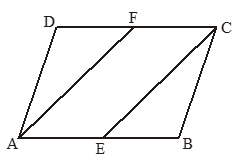1. 1/2
2. 2
3. 1
4. 4

2. In the given rectangle ABCD, AB : BC =√3 : 2 in circle touching only sides AB and CD, while circumcircle passing through all vertex. Find ratio of strips and cross-shaded area :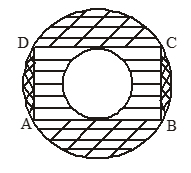1. 2(11-4√3)/7(14√3-22)
2. 2(14√3-22)/7(11-4√3)
3. 2/7
4. 7/2 ((7√3-11)/(12-2√3))

3. Find ratio of area of ΔADF and ΔABE, F and E are such that

DF = FC, 2BE = EC, ABCD is parallelogram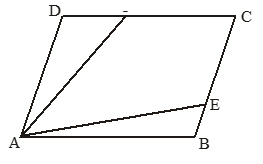1. 3
2. 5/2
3. 2
4. 3/2

4. ΔABCD is trapezium if AB = 20 cm, CD = 15cm, DE  AB, CF  AB if Ar(ΔADE)/Ar(ΔCFB) =3/4.

Find FB – AE.

1. 3/7
2. 4/7
3. 5/7
4. 6/7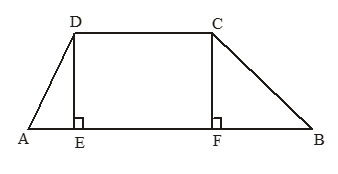5. In given ΔABC

DE || FG || BC

Find ratio of area ΔADE and FGCB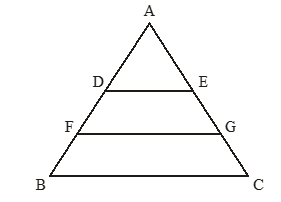1. 1/4
2. 1/5
3. 1/6
4. 1/7

6. In given figure ABCD is parallelogram. If AE = 2AD and

AF = 2DG = AB/2 .

What will ratio of area of ΔDEG and quadrilateral GCBF.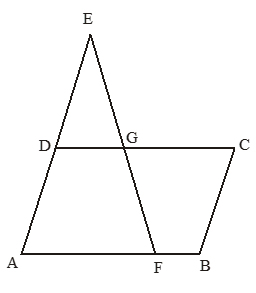1. 1/5
2. 1/6
3. 1/4
4. 1/3

7. Quadrilateral ABCD is formed by unfolding a circle of same perimeter as ABCD. What is ratio of maximum area possible of quadrilateral and circle’s area ?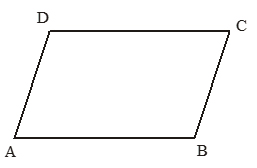1. π/2
2. π/3
3. π/4
4. π/5

8. In given figure a sheet of dimension 20 x 30 is given. If from each corner a square of 5 cm side was cut. Find the volume of parallel piped formed by folding sides (as shown in fig.)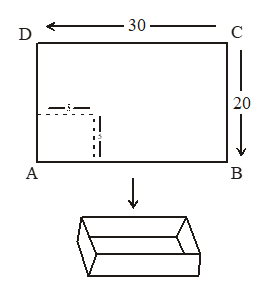1. 1000 cm3
2. 500 cm3
3. 2000 cm3
4. 3000 cm3

9. Form a rectangle of dimensions a rhombus is formed by joining midpoint of each side. Find the ration of area of rhombus and remains part of rectangle.

1. 1/2
2. 1
3. 3/2
4. 2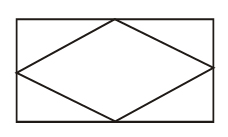10. For a fire length of perimeter, what is correct order of area of different shapes ?

1. Circle>Square>Rectanle
2. Circle>Rectanle >Square
3. Rectanle >Circle>Square
4. Rectanle > Square>Circle

11. Find the area of Rectangle ABCD. It is given that arc of circle passes through point A, D and touches BC at midpoint. Radius of circle in 13 cm & BC = 24 cm.

1. 96 cm2
2. 384 cm2
3. 144 cm2
4. 192 cm2

12. In given fig. ABCD is a rectangle .A small circle C1of radius R1 touches side DC at mid point E. AFB is a part of circle (c2) of radius R2 . EF =BC/6 .(at point F both circles touches each other).If Find R2 – R1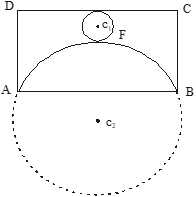1. 26 cm
2. 25 cm
3. 24 cm
4. 23 cm

Solutions: to worksheet-9 Topic-Area of Quadrilateral(parallelogram)

 1. (a) 2. (b) 3. (d) 4. (c) 5. (b) 6. (a) 7. (c) 8. (a) 9. (b) 10. (a) 11. (d) 12. (c)